•IG算法
• 一般地，一个决策树包含一个根节点，若干个内部节点和若干个叶节点，叶结点对应决策结果，其他每个节点对应于一个属性...信息信息熵是度量样本集合纯度最常用的一种指标，假定当前样本集合D中第k类样本所占比例...

一般地，一个决策树包含一个根节点，若干个内部节点和若干个叶节点，叶结点对应决策结果，其他每个节点对应于一个属性测试，每个结点包含的样本集合根据属性测试的结果被划分到子节点中；根节点包含样本全集，从根节点到每个叶节点的路径对应了一个判定测试序列。决策树学习的目的是为了产生一颗泛化能力强，处理未见实例能力强的决策树。

信息熵

信息熵是度量样本集合纯度最常用的一种指标，假定当前样本集合D中第k类样本所占比例为pk(1,2,..|y|),则D的信息熵定义为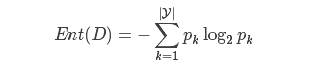其中Ent(D)的值越小，则D的纯度越高。

信息增益

假定离散属性a有V个可能的取值a1,a2,…,aV,若使用a来对样本集D进行划分，则会产生V个分支节点，其中第v个分支节点包含了D中所有在属性a上取值为av的样本，记为Dv,根据信息熵的公式，在考虑到不同的分支节点所包含的样本数不同，给分支节点赋予权重|Dv|/|D|,即样本数越多的分支节点的影响越大，于是可计算出用属性a对样本集D进行划分所获得的”信息增益”。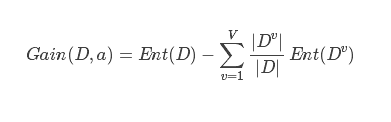一般而言，信息增益越大，则意味着使用属性a来进行划分所得的”纯度提升”越大。因此，我们可以用信息增益来进行决策树的划分属性选择。

实例分析

以下表的数据为例：其中有17个训练正例，学习目标是预测是不是好瓜的决策树。分类目标|Y|=2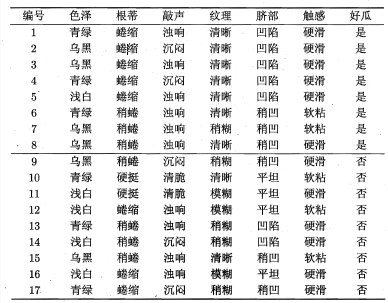信息熵计算得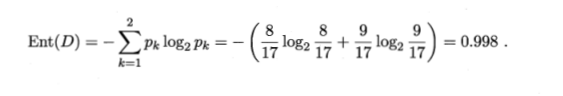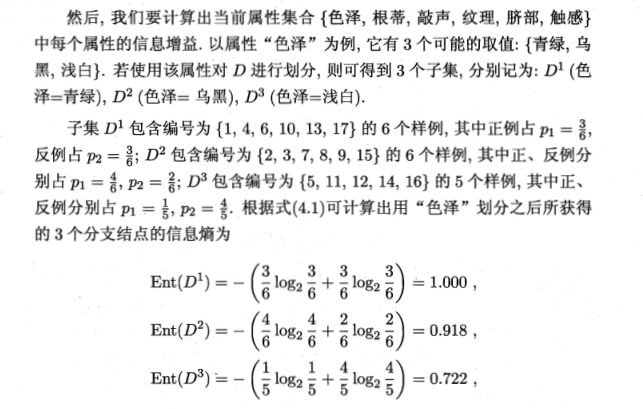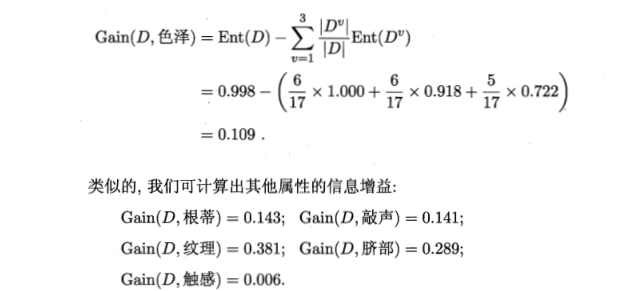上述实现代码为

close all;

clear all;

clc;

InforGain = gain(data);

function InforGain = gain(data)

[m, n] = size(data);

InforGain = zeros(n-1,2);

labels = data(:,n);

for i=1:n

tmp{i} = [];

percen{i} = [];

col = data(:,i);

unicol = unique(col);

%计算每一列有几类，并把每一类的信息熵和比例存储起来

for j = 1:length(unicol)

num = length(find(col==unicol(j)));

pnum = length(find(col==unicol(j) & labels == 1));

rate = pnum/num;

if i==7

rate = num/length(labels);

end

gain = -(rate*log2(rate)+(1-rate)*log2(1-rate));

tmp{i}=[tmp{i} gain];

percen{i}=[percen{i} num/length(col)];

end

end

%整体信息熵

InforEntropy = tmp{length(tmp)}(1);

%将NAN转化为0

for i = 1:length(tmp)

tmp{i}(isnan(tmp{i})) = 0;

%disp(tmp{i});

end

%求每一个属性列的信息增益

for i = 1:length(percen)-1

InforGain(i,:) = [i,roundn(InforEntropy-sum(tmp{i}.*percen{i}),-3)];

disp(InforEntropy-sum(tmp{i}.*percen{i}));

end

作者：听城

展开全文• matlab开发-Matlabcode中的增益算法。高效的纯matlab图形算法实现，以补充matlabbgl的mex函数。
• 基于信息增益的决策树算法（附MATLAB代码）

千次阅读 热门讨论 2019-12-18 11:48:47
基于信息增益的决策树算法（附MATLAB代码） 最近在学机器学习，本篇文章的内容正好是作业内容，所以拿来分享一下，顺便捋一下思路。下面内容只涉及到决策树学习基本算法(伪代码)、信息增益的计算和matlab代码实现。...

基于信息增益的决策树算法（附MATLAB代码）

最近在学机器学习，本篇文章的内容正好是作业内容，所以拿来分享一下，顺便捋一下思路。下面内容只涉及到决策树学习基本算法(伪代码)、信息增益的计算和matlab代码实现。决策树算法原理不再赘述，请自行百度。水平有限，如有错误，欢迎指正！

一、决策树学习基本算法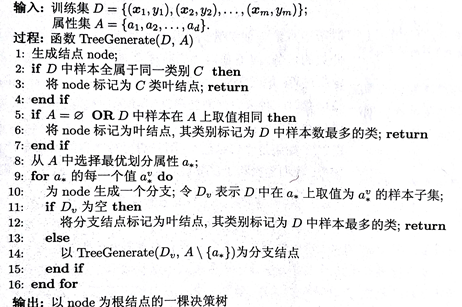二、 信息增益的计算

1.信息熵

“信息熵”（information entropy）是度量样本集合纯度最常用的一种指标，假定当前样本集合D中第k类样本所占的比例为Pkk = 1,2,…,|Y|），则D的信息熵定义为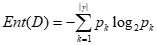Ent(D)的值越小，D的纯度越高。

2.信息增益

假定离散属性aV个可能的值a1,a2,…,aV，若使用a来对样本集D进行划分，则会产生V个分支节点，其中第v个分支节点包含了D中所有在属性a上取值为av的样本，记为Dv，这时可以计算出Dv的信息熵，同时考虑到不同的分支结点所包含的样本数不同，给分支结点赋予权重|Dv|/|D|，即样本数越多的分支结点的影响越大，于是可计算出用属性a对样本集D进行划分所获得的“信息增益”（information gain）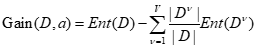3.划分属性选择

一般而言，信息增益越大，则意味着使用属性a来进行划分所获得的“纯度提升”就越大，因此，我们可用信息增益来进行决策树的划分选择，即选择属性a = arg* max Gain(D,a)，这就是著名的ID3决策树学习算法。

三、MATLAB代码实现

%****************************************
%main.m
%****************************************
clear,clc
Node = createTree(data, feature);       %生成决策树
drawTree(Node)                          %绘制决策树

%****************************************
%createTree.m
%****************************************
%生成决策树ID3算法
%data：训练集
%feature：属性集
function [node] = createTree(data, feature)
type = mostType(data);  %cell类型
[m, n] = size(data);
%生成节点node
%value：分类结果，若为null则表示该节点是分支节点
%name：节点划分属性
%branch：节点属性值
%children：子节点
node = struct('value','null','name','null','branch','null','children',{});
temp_type = data{1, n};
temp_b = true;
for i = 1 : m
if temp_type ~= data{i, n}
temp_b = false;
end
end
%样本中全为同一分类结果，则node节点为叶节点
if temp_b == true
node(1).value = data(1, n); %cell类型
return;
end
%属性集合为空，将结果标记为样本中最多的分类
if isempty(feature) == 1
node.value = type;  %cell类型
return;
end
%获取最优划分属性
feature_bestColumn = bestFeature(data); %最优属性列数，double类型
best_feature = data(:,feature_bestColumn); %最优属性列，cell类型
best_distinct = unique(best_feature); %最优属性取值
best_num = length(best_distinct); %最优属性取值个数
best_proc = cell(best_num, 2);
best_proc(:, 1) = best_distinct(:, 1);
best_proc(:, 2) = num2cell(zeros(best_num, 1));
%循环该属性的每一个值
for i = 1:best_num
%为node创建一个bach_node分支，设样本data中该属性值为best_proc(i, 1)的集合为Dv
bach_node = struct('value', 'null', 'name', 'null', 'branch', 'null', 'children',{});
Dv_index = 0;
for j = 1:m
if data{j, feature_bestColumn} == best_proc{i, 1}
Dv_index = Dv_index + 1;
end
end
Dv = cell(Dv_index, n);
Dv_index2 = 1;
for j = 1:m
if best_proc{i, 1} == data{j, feature_bestColumn}
Dv(Dv_index2, :) = data(j, :);
Dv_index2 = Dv_index2 + 1;
end
end
Dfeature = feature;
%Dv为空则将结果标记为样本中最多的分类
if isempty(Dv) == 1
bach_node.value = type;
bach_node.name = feature(feature_bestColumn);
bach_node.branch = best_proc(i, 1);
node.children(i) = bach_node;
return;
else
Dfeature(feature_bestColumn) = [];
Dv(:,feature_bestColumn) = [];
%递归调用createTree方法
bach_node = createTree(Dv, Dfeature);
bach_node(1).branch = best_proc(i, 1);
bach_node(1).name = feature(feature_bestColumn);
node(1).children(i) = bach_node;
end
end
end

%****************************************
%mostType.m
%****************************************
%计算样本最多的结果
function [res] = mostType(data)         %返回值cell类型
[m,n] = size(data);
res = data(:, n);
res_distinct = unique(res);
res_num = length(res_distinct);
res_proc = cell(res_num,2);
res_proc(:, 1) = res_distinct(:, 1);
res_proc(:, 2) = num2cell(zeros(res_num,1));
for i = 1:res_num
for j = 1:m
if res_proc{i, 1} == data{j, n};
res_proc{i, 2} = res_proc{i, 2} + 1;
end
end
end
end

%****************************************
%getEntropy.m
%****************************************
%计算信息熵
function [entropy] = getEntropy(data)       %返回值double类型
entropy = 0;
[m,n] = size(data);
label = data(:, n);
label_distinct = unique(label);
label_num = length(label_distinct);
proc = cell(label_num,2);
proc(:, 1) = label_distinct(:, 1);
proc(:, 2) = num2cell(zeros(label_num, 1));
for i = 1:label_num
for j = 1:m
if proc{i, 1} == data{j, n}
proc{i, 2} = proc{i, 2} + 1;
end
end
proc{i, 2} = proc{i, 2} / m;
end
for i = 1:label_num
entropy = entropy - proc{i, 2} * log2(proc{i, 2});
end
end

%****************************************
%getGain.m
%****************************************
%计算信息增益
function [gain] = getGain(entropy, data, column)        %返回值double类型
[m,n] = size(data);
feature = data(:, column);
feature_distinct = unique(feature);
feature_num = length(feature_distinct);
feature_proc = cell(feature_num, 2);
feature_proc(:, 1) = feature_distinct(:, 1);
feature_proc(:, 2) = num2cell(zeros(feature_num, 1));
f_entropy = 0;
for i = 1:feature_num
feature_row = 0;
for j = 1:m
if feature_proc{i, 1} == data{j, column}
feature_proc{i, 2} = feature_proc{i, 2} + 1;
feature_row = feature_row + 1;
end
end
feature_data = cell(feature_row,n);
feature_row = 1;
for j = 1:m
if feature_distinct{i, 1} == data{j, column}
feature_data(feature_row, :) = data(j, :);
feature_row = feature_row + 1;
end
end
f_entropy = f_entropy + feature_proc{i, 2} / m * getEntropy(feature_data);
end
gain = entropy - f_entropy;
end

%****************************************
%bestFeature.m
%****************************************
%获取最优划分属性
function [column] = bestFeature(data)       %返回值double类型
[~,n] = size(data);
featureSize = n - 1;
gain_proc = cell(featureSize, 2);
entropy = getEntropy(data);
for i = 1:featureSize
gain_proc{i, 1} = i;
gain_proc{i, 2} = getGain(entropy, data, i);
end
max = gain_proc{1,2};
max_label = 1;
for i = 1:featureSize
if gain_proc{i, 2} >= max
max = gain_proc{i, 2};
max_label = i;
end
end
column = max_label;
end

%****************************************
%drawTree.m
%****************************************
% 画出决策树
function [] = drawTree(node)
% 遍历树
nodeVec = [];
nodeSpec = {};
edgeSpec = [];
[nodeVec,nodeSpec,edgeSpec,~] = travesing(node,0,0,nodeVec,nodeSpec,edgeSpec);
treeplot(nodeVec);
[x,y] = treelayout(nodeVec);
[~,n] = size(nodeVec);
x = x';
y = y';
text(x(:,1),y(:,1),nodeSpec,'FontSize',15,'FontWeight','bold','VerticalAlignment','bottom','HorizontalAlignment','center');
x_branch = [];
y_branch = [];
for i = 2:n
x_branch = [x_branch; (x(i,1)+x(nodeVec(i),1))/2];
y_branch = [y_branch; (y(i,1)+y(nodeVec(i),1))/2];
end
text(x_branch(:,1),y_branch(:,1),edgeSpec(1,:),'FontSize',12,'Color','blue','FontWeight','bold','VerticalAlignment','bottom','HorizontalAlignment','center');
end

% 遍历树
function [nodeVec,nodeSpec,edgeSpec,current_count] = travesing(node,current_count,last_node,nodeVec,nodeSpec,edgeSpec)
nodeVec = [nodeVec last_node];
if isempty(node.value)
nodeSpec = [nodeSpec node.children(1).name];
else
if strcmp(node.value, '是')
nodeSpec = [nodeSpec '好瓜'];
else
nodeSpec = [nodeSpec '坏瓜'];
end
end
edgeSpec = [edgeSpec node.branch];
current_count = current_count + 1;
current_node = current_count;
if ~isempty(node.value)
return;
end
for next_ndoe = node.children
[nodeVec,nodeSpec,edgeSpec,current_count] = travesing(next_ndoe,current_count,current_node,nodeVec,nodeSpec,edgeSpec);
end
end

代码链接：.m文件下载

四、运行结果

训练集：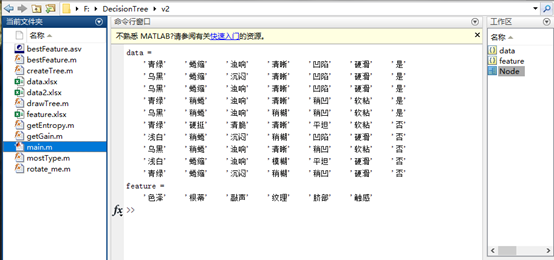根据训练集生成决策树如下：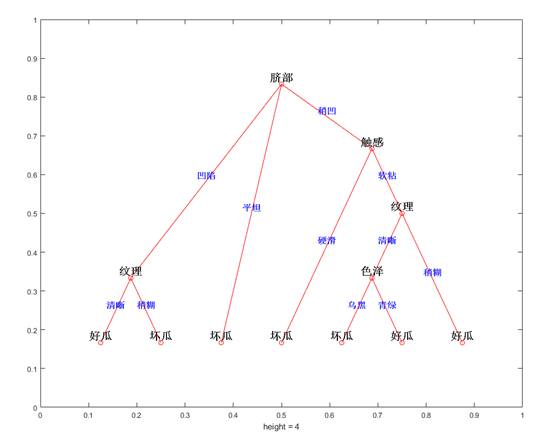测试集及结果：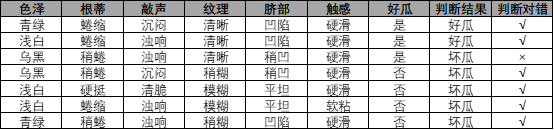声明：本人并没有写测试部分的代码，感兴趣的可以自己写一下。

五、代码和数据集

展开全文决策树 matlab
• 自动增益控制 (AGC) 算法用于自动调整音频信号的语音电平。matlab 输入和输出信号范围从 -1 到 +1（1.1 版用于单声道和立体声输入）和浮点 C 代码（仅限对于单声道）输入和输出值的范围从 -32768 到 +32767（即通常...matlab
• 提出了基于信息增益率的用电客户指标选择方法,根据信息增益率的大 小选择对...matlabcode. 2·Proposing a new way based on information-gain-ratio to ......(A,Ts) 3 求控制系统的增益和传递零点求系统增益的...

2。提出了基于信息增益率的用电客户指标选择方法,根据信息增益率的大 小选择对...matlabcode. 2·Proposing a new way based on information-gain-ratio to ......

(A,Ts) 3 求控制系统的增益和传递零点求系统增益的MATLAB函数: K=dcgain(num,den) K=dcgain(A,B,C,D) 7.2 控制系统的稳定性分析 1 利用极点判断系统......

双击打开模 块窗口 取信号源Sources/Step 双击可弹出属性窗 口编辑属性弹开右边模 块窗口 鼠标选中后拖拽 到仿真窗口 取增益Math/Gain 双击可弹出属性窗 口编辑......

运用放大电路的高频 模型,通过对之流通路和交流通路的求解计算出放大电路的增益函数,并用 Matlab 画出了该放大电路 的幅度相应和相位响应,在 Multisim 软件中进行......

单击MATLAB Command窗口工具条上的Simulink图标,或者在...Continuous 库 No Image :零极点增益形式的传递函数...Gain 100 Gain1 7.4 应用举例 模块参数设置: ......

如果要想在仿真阶段修改增益值,可以使用 slider gain模块。 数据类型 依赖于用户...第3章 MATLAB与Simulink... 35页 免费 simulink matlab教程 36页 1下载券 ......

第十讲 MATLAB的SIMULINK仿真 10.1 10.2 10.3 10.4 10.5 10.6 Simulink...1 In 1 Gain 1 Out ? 用 mdl 文件编写有输入和输出的增益器。 (在 Word......

第四章 控制系统 MATLAB 仿真基础 § 4.1 控制系统的数学模型 1、 2、 3、 4、 、 传递函数模型(tranfer function) 零极点增益模型(zero-pole-gain) 状态......

MATLAB语言 Matrix Gain MinMax Product Real-Image to Complex 矩阵增益 求输入的最大或最小值 乘积 将实部和虚部合成复数 Relational Operator Reshape Rounding ......

MATLAB70使用详解-第10章 SIMULINK高级仿真技术 10....以便这些变量 在被封子系统模块图标绘制指令或其他...? 改变封装子系统参数设置对话框中 Gain 和 ......

转角频率和渐近线 计算转角频率,并使用 MATLAB 画出例 6 伯德图的幅频特性...(mag),'--') hold on lfg=dcgain(G) cf1_dB=20*log10(lfg) plot([......

传递函数模型(系统的外部模型); ? 状态方程模型(系统的内部模型); ? 零极点增益模型和部分分式模型等 MATLAB中控制系统 的数学描述与建模在线性系统理论中,一般......

四、零极点增益模型转换为状态空间、传递函数模型 1、MATLAB 算法 %将零极...

MATLAB基础及工程应用 2014 年 11 月 6 日 8 / 44 Simulink基础 5) Math(数学模块) Sum: 加减运算 Product: 乘运算 Dot Product: 点乘运算 Gain: 比例运算......

在 MATLAB中,专门提供了绘制根轨迹的有关函数。 pzmap:绘制线性系统的零极点图 rlocus:求系统根轨迹。 rlocfind:计算给定一组根的根轨迹增益。 sgrid:在连续系统......

(零极点增益模型) 一、数学模型的建立(零极点增益模型 2、零极点增益(Zero-Pole-Gain:ZPK)模型 、零极点增益( ) G (s) = K ∏(s ? z ) ∏(s ? p......

在MATLAB中,专门提供了 绘制根轨迹的有关函数。 rlocus:求系统根轨迹。 rlocfind:计算给定一组根的根轨迹增益。 对于图所示的负反馈系统,其特征方程可表示为 1 ......

在 MATLAB中,专门提供了绘制根轨迹的有关函数。 pzmap:绘制线性系统的零极点图 rlocus:求系统根轨迹。 rlocfind:计算给定一组根的根轨迹增益。 sgrid:在连续系统......

模块 Sum Product Dot Product Gain Slider Gain Matrix Gain Math Function ...常数增益 可变增益 矩阵增益 数学运算函数 三角函数 求最大值 求绝对值 符号......

展开全文matlab增益处理
•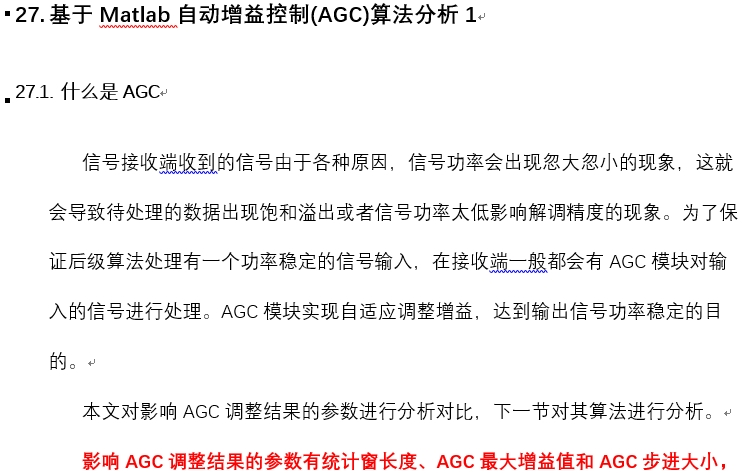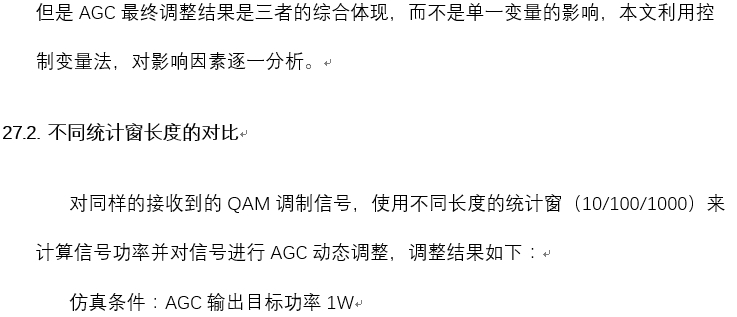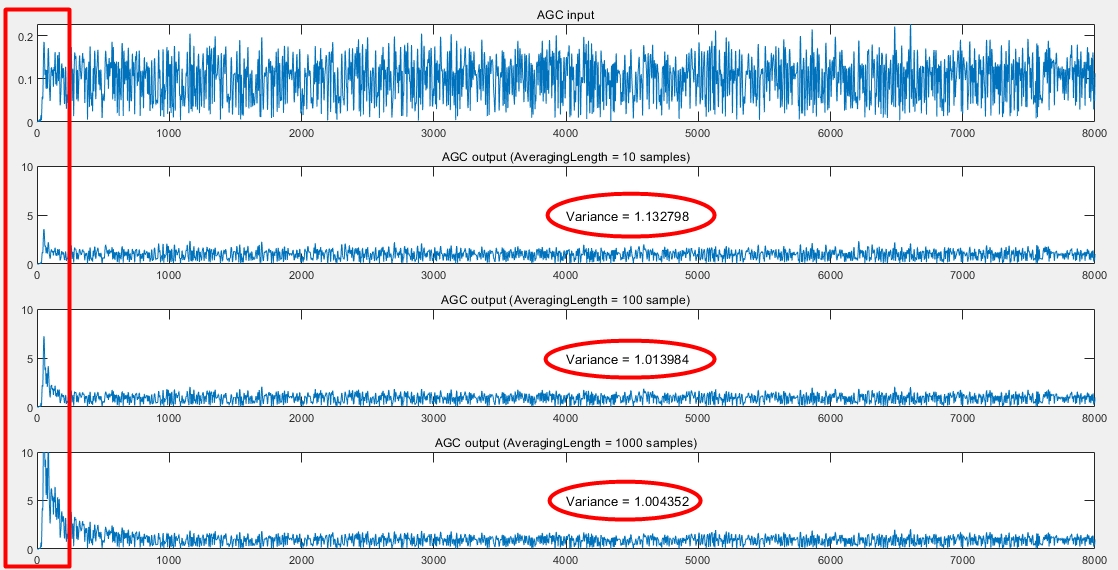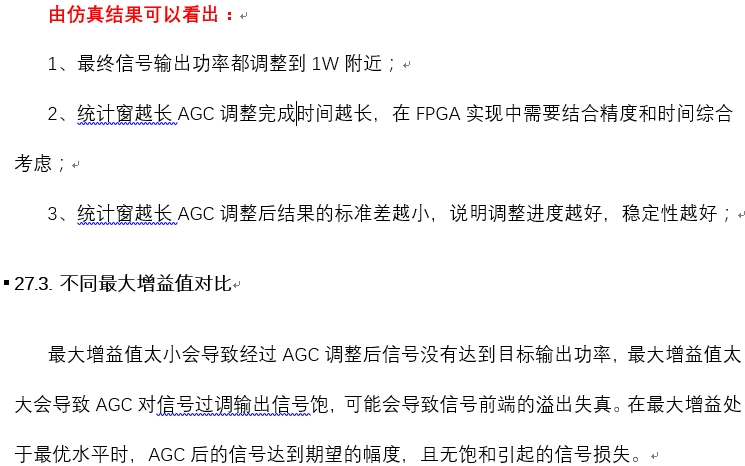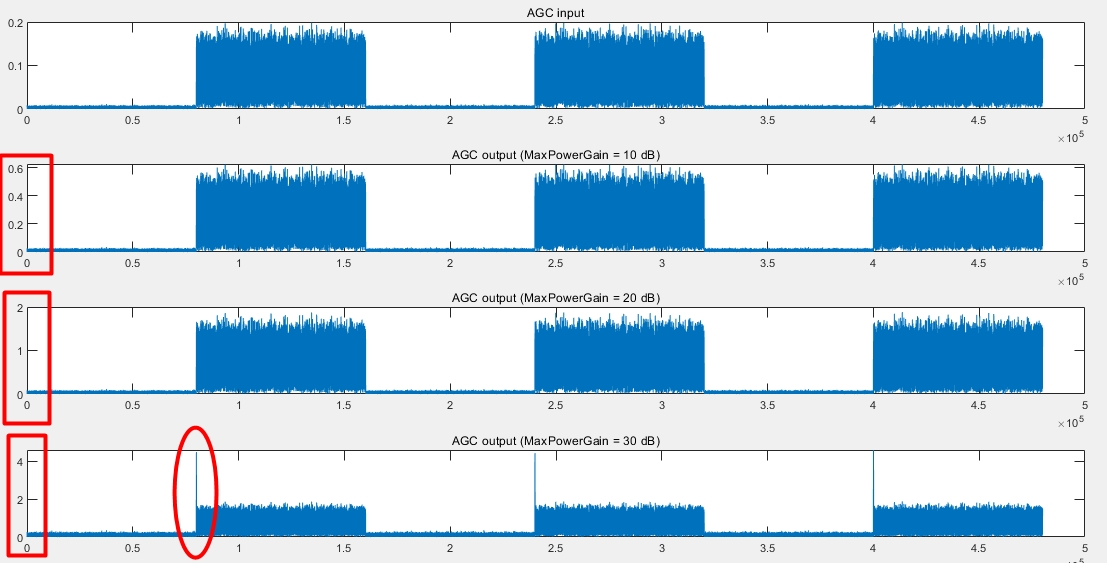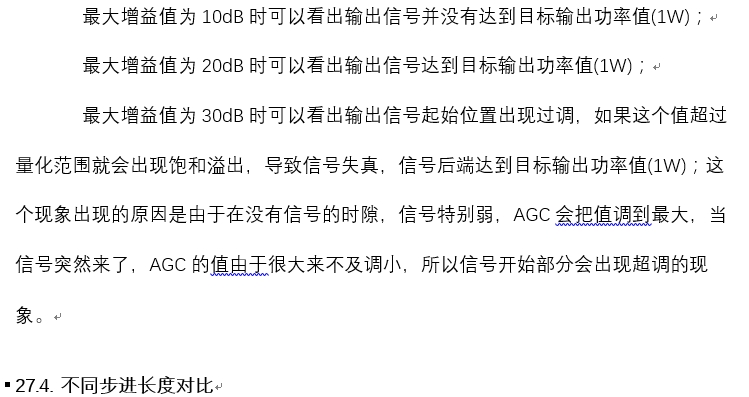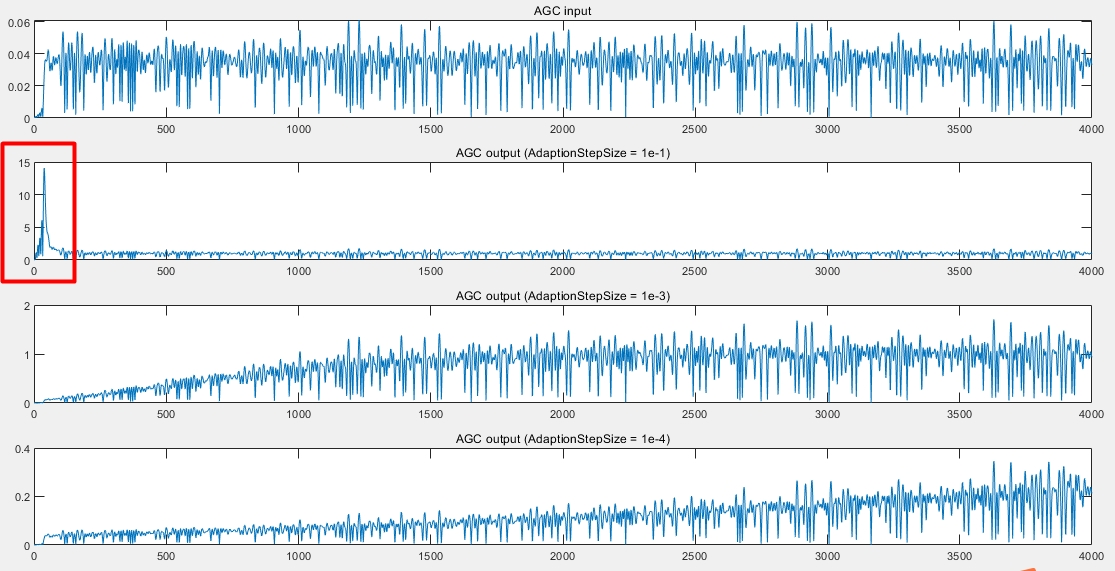展开全文• 保守值法matlab代码如果您使用此存储库中提供的资源（算法，代码和数据集），请引用我们的论文。 *此页面底部提供了BibTeX条目。 （IGTS）多元时间序列的时间分割 基于信息增益的指标，用于识别人类活动的转变 这项...
• 使用数据集 西瓜数据集3共8属性 6离散2连续 离散属性用1，2，3代表不同的取值 因为实在不太会用Matlab处理文本(真想...所以只能用matlab自带的树形结构，只有形状，具体信息还是要去看代码运行的结果，不过重点是明...
• 因为这时概率分布的信息熵最大，所以称之为“最大熵法”。最大熵法在数学形式上很漂亮，但是实现起来比较复杂，但把它运用于金融领域的诱惑也比较大，比如说决定股票涨落的因素可能有几十甚至上百种，而最大熵方法...
• 利用Matlab编制一个遗传算法或粒子群算法程序，并实现对间距为半波长均匀直线阵综合，指标如下： 阵元数：16元 副瓣电平： 增益：>11dB 要求撰写设计报告，内容包括：所采用的算法基本原理，目标函数的设计，各个...
• 使用数据集 西瓜数据集3共8属性 6离散2连续 离散属性用1，2，3代表不同的取值 因为实在不太会用Matlab处理文本(真想...所以只能用matlab自带的树形结构，只有形状，具体信息还是要去看代码运行的结果，不过重点是明...
•  %计算卡尔曼增益 7 V! z6 k0 f8 A e = Z(k) - H*X_pre; %新息 本次测量值与上次预测值之差 " A7 Q y/ F* q6 g5 [% O% _1 E Xkf(k) = X_pre + Kg*e; %状态更新 本次预测值# }7 B- k9 r4 W; ~9 f P(k) = (I -...
• matlab算法集锦

2021-09-06 19:15:25
算法集锦 决策树-划分点 function [n,h]=huafendian1(x) %n返回增益 %h返回划分点 %假设0代表第一类 %假设1代表第二类 %输入x第一列为属性，第二列为用于学习的分类结果 [~,m]=sort(x(:,1));%按小到大排序 x=x(m,:)...matlab 算法
• 本练习设计了一个基于语音波形的短时间估计的语音波形自动增益控制系统。 语音信号方差（或等效的短时标准偏差）。 AGC 系统以音节速率（对短时语音信号方差变化的缓慢响应）或瞬时速率（对短时语音信号方差变化的...matlab
• 浅谈决策树算法以及matlab实现ID3算法

万次阅读 多人点赞 2016-02-27 00:04:10
在20世纪70年代后期和80年代初期，机器学习研究者J.Ross Quinilan提出了ID3算法以后，决策树在机器学习、数据挖掘领域得到极大的发展。Quinilan后来又提出了C4.5，成为新的监督学习算法。1984年几位统计学家提出了...matlab 机器学习 数据挖掘 ID3算法
• 利用Matlab编制一个遗传算法或粒子群算法程序，并实现对间距为半波长均匀直线阵综合，指标如下： 阵元数：16元 副瓣电平： 增益：>11dB 要求撰写设计报告，内容包括：所采用的算法基本原理，目标函数的设计，各个...
•matlab 数学建模 线性代数 矩阵 算法
• 用于计算系统状态的卡尔曼最优增益和最小均方误差 (MMSE) 估计的代码。 一阶和二阶模型的示例。 可轻松适应其他系统和输入，非常适合学习应用。matlab
• 卡尔曼滤波器是1种利用线性系统状态方程，通过系统输入输出观测数据，对系统状态进行最优估计的算法。而且由于观测包括系统的噪声和干扰的影响，所以最优估计也可看作是滤波进程。卡尔曼滤波器的核心内容就是5条公式...
• Matlab常用白平衡算法》由会员分享，可在线阅读，更多相关《Matlab常用白平衡算法(21页珍藏版)》请在人人文库网上搜索。1、1 灰色世界法灰色世界法(grey world method) 要计算未知光源的特性必须从图片中提取相关...
• matlab中存档算法代码空房间 与空房间项目相关的研究代码。 文件夹布局： GAPS：普林斯顿大学汤姆·冯克豪斯（Tom Funkhouser）的GAPS几何图形库。 包含各种网格和3D几何例程和数据结构此版本基于COS526中使用的版本...
• 传递函数、零极点增益与状态空间三种模型转换的MATLAB算法实现一、引言微分方程是自控控制系统最原始的数学模型，它反映系统动态运行规律。时域分析中要用拉普拉斯变换定义传递函数，再做其它转化。为了方便我们对...
• LPC语音信号算法MATLAB实现 为什么选择LPC？ 在通信系统中，由于通道的带宽限制，通常有必要以压缩或编码形式传输音频（语音）信号。 在这方面，“线性预测编码（LPC）”是一种低比特率的语音编码的有效方法。 ...
• 1引言在水声换能器工作时,单个点源基元是无方向性的,将多个换能器基元按...相比于无指向性的点源,声基阵在指定方向上信噪比的提高称为阵增益,它是衡量声基阵性能的重要指标,也是声纳方程的重要构成。阵增益AG(Array ...matlab做阵型图
• 传递函数零极点增益与状态空间转换的matlab算法实现传递函数、零极点增益与状态空间三种模型转换的MATLAB算法实现一、引言微分方程是自控控制系统最原始的数学模型，它反映系统动态运行规律。时域分析中要用拉普拉斯...
• 差分进化算法概述 20世纪60年代初，美国 Michigan大学的.Holland教授借鉴与生物进化机制提出了自适应机器人学习，即成为后来广受人们关注的遗传算法； I Rechenberg和 LL.Foge等人都面临着各自研究领域所遇到的复杂...matlab
• Chow算法是根据各个子信道的信道容量来分配比特的。它的优化准则是在维持目标误比特率的前提下，使系统的余量最大。该算法通过迭代过程，逐步分配比特，同时使系统的余量逐步增大，直到所有的比特都分配完毕。 比特...matlab 算法 开发语言
• 因此，今天笔者想要分享的是随机森林算法在岩性识别中的应用与代码实现。 科普中国·科学百科定义：随机森林（Random forest）指的是利用多棵树对样本进行训练并预测的一种分类器。 通俗地来讲，随机森林算法从...算法 matlab 随机森林...

matlab信息增益算法matlab 订阅